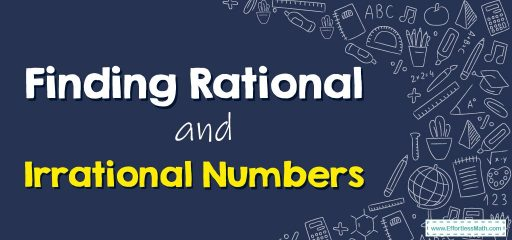# How to Find Rational and Irrational Numbers?

Rational and Irrational numbers both are real numbers but different in their properties. This guide will teach you about rational and irrational numbers and their properties.A rational number contains any whole number, fraction, or decimal that ends or repeats. An irrational number is any number that cannot be converted to a fraction, so any number that does not match the definition of a rational number.

## A step-by-step guide torational and irrational numbers

Rational numbers are terminating or non-terminating repeating numbers, while irrational numbers are those that do not end or repeat after a certain number of decimal places.

### Irrational numbers

Irrational numbers are a set of real numbers that cannot be expressed as fractions, $$\frac{p}{q}$$ where $${p}$$ and $${q}$$ are integers. The denominator $$q$$ is not equal to zero $$(q ≠ 0)$$. Also, the decimal expansion of an irrational number is neither terminated nor repeated. The set of irrational numbers is represented by $$Q´$$.

### Properties of irrational numbers

Irrational number properties help us select irrational numbers from a set of real numbers. The following are some characteristics of irrational numbers:

• Irrational numbers consist of non-terminating and non-recurring decimals.
• These are real numbers only.
• When an irrational number and a rational number are added, the result of some of them is just an irrational number. For an irrational number $$x$$ and a rational number $$y$$, the result is $$x + y =$$ an irrational number.
• When any irrational numbers are multiplied by any nonzero rational number, their product is an irrational number. For an irrational number $$x$$ and a rational number y, their product is $$xy=$$ irrational.
• For any two irrational numbers, their least common multiple $$(LCM)$$ may or may not exist.
• Addition, subtraction, multiplication, and division of two irrational numbers may or may not be rational numbers.

### How to identify an irrational number?

We know that irrational numbers are just real numbers that cannot be expressed as $$\frac{p}{q}$$, where $$p$$ and $$q$$ are integers and $$q ≠ 0$$. For example, $$\sqrt{5}$$ and $$\sqrt{3}$$ are irrational numbers. On the other hand, the numbers can be represented as $$\frac{p}{q}$$, such that $$p$$ and $$q$$ are integers and $$q≠0$$ are rational numbers.

### Rational numbers

A rational number is a number that is of the form $$\frac{p}{q}$$ where $$p$$ and $$q$$ are integers and $$q$$ is not equal to $$0$$. The set of rational numbers is denoted by $$Q$$. In other words, if a number can be expressed as a fraction in which both the numerator and the denominator are integers, that number is rational.

### How to identify rational numbers?

To find out if a given number is a rational number, we can check whether it corresponds to any of these conditions:

• We can represent the given number as a fraction of integers.
• We the decimal expansion of the number is terminating or non-terminating repeating.
• All whole numbers are rational numbers.

### Rational and Irrational Numbers – Example 1:

Classify the following as rational and irrational numbers. $$[e, -5, \sqrt{9},\sqrt{13}, π, -\frac {2}{8}]$$

Solution:

$$-5$$ is an integer, $$\sqrt{9}$$ is a perfect square, and $$-\frac{2}{8}$$ has a recurring terminating decimal value. These numbers are rational numbers. The irrational numbers are $$e,\sqrt{13}, π$$.

## Exercises forRational and Irrational Numbers

### Classify the following numbers as rational and irrational numbers.

1. $$\color{blue}{\sqrt{12}}$$
2. $$\color{blue}{\frac{22}{8}}$$
3. $$\color{blue}{0}$$
4. $$\color{blue}{2.23123123412}$$
1. $$\color{blue}{Irrational\:number}$$
2. $$\color{blue}{Rational\:number\:}$$
3. $$\color{blue}{Rational\:number\:}$$
4. $$\color{blue}{Irrational\:number}$$

### What people say about "How to Find Rational and Irrational Numbers? - Effortless Math: We Help Students Learn to LOVE Mathematics"?

No one replied yet.

X
30% OFF

Limited time only!

Save Over 30%

SAVE $5 It was$16.99 now it is \$11.99